# Variational Autoencoder with Tensorflow 2.8 – III – problems with the KL loss and eager execution

In the last posts of this series

I have discussed basics of Autoencoders. We have also set up a simple Autoencoder with the help of the functional Keras interface to Tensorflow 2. This worked flawlessly and we could apply our Autoencoder [AE] to the MNIST dataset. Thus we got a good reference point for further experiments. Now let us turn to the design of “Variational Autoencoders” [VAEs].

In the present post I want to demonstrate that some simple classical recipes for the construction of VAEs may not work with Tensorflow [TF] > version 2.3 due to “eager execution mode”, which is activated as the default environment for all command interpretation and execution. This includes gradient determination during the forward pass through the layers of an artificial neural network [ANN]. In contrast to “graph mode” for TF 1.x versions.

Addendum 25.05.2022: This post had to be changed as its original version contained wrong statements.

As we know already form the first post of this series we need a special loss function to control the parameters of distributions in the latent space. These distributions are used to calculate z-points for individual samples and must be “fit” optimally. I list four methods to calculate such a loss. All methods are taken form introductory books on Machine Learning (see the book references in the last section of this post). I use one concrete and exemplary method to realize a VAE: We first extend the layers of the AE-Encoder by two layers (“mu”, “var_log”) which give us the basis for the calculation of z-points on a statistical distribution. Then we use a special layer on top of the Decoder model to calculate the so called “Kullback-Leibler loss” based on data of the “mu” and “var_log” layers. Our VAE Keras model will be based on the Encoder, the Decoder and the special layer. This approach will give us a typical error message to think about.

# Building a VAE

A VAE (as an AE) maps points/vectors of the “variable space” to points/vectors in the low-dimensional “latent space”. However, a VAE does not calculate the “z-points” directly. Instead it uses a statistical variable distribution around a mean value. This opens up for further degrees of freedom, which become subjects to the optimization process. These degrees of freedom are a mean value “mu” of a distribution and a “standard deviation”. The latter is derived from a variance “var“, of which we take the logarithm “log_var” for practical and numerical reasons.

Note: Our neural network parts of the VAE will decide themselves during training for which samples they use which mu and which var_log values. They optimize the required values via specific weights at special layers.

# A special function

We first define a function whose meaning will become clear in a minute:

```# Function to calculate a z-point based on a Gaussian standard distribution
def sampling(args):
mu, log_var = args
# A randomized value from a standard distribution
epsilon = B.random_normal(shape=B.shape(mu), mean=0., stddev=1.)
# A point in a Gaussian standard distribution defined by mu and var with log_var = log(var)
return mu + B.exp(log_var / 2) * epsilon
```

This function will be used to calculate z-points from other variables, namely mu and log_var, of the Encoder.

# The Encoder

The VAE Encoder looks almost the same as the Encoder of the AE which we build in the last post:

```z_dim = 16

# The Encoder
# ************
encoder_input = Input(shape=(28,28,1))
x = encoder_input
x = Conv2D(filters = 32, kernel_size = 3, strides = 1, padding='same')(x)
x = LeakyReLU()(x)
x = Conv2D(filters = 64, kernel_size = 3, strides = 2, padding='same')(x)
x = LeakyReLU()(x)
x = Conv2D(filters = 128, kernel_size = 3, strides = 2, padding='same')(x)
x = LeakyReLU()(x)

# some information we later need for the decoder - for MNIST and our layers (7, 7, 128)
shape_before_flattening = B.int_shape(x)[1:]  # B is the tensorflow.keras backend ! See last post.

x = Flatten()(x)of

# differences to AE-models. The following layers central elements of VAEs!
mu      = Dense(z_dim, name='mu')(x)
log_var = Dense(z_dim, name='log_var')(x)

# We calculate z-points/vectors in the latent space by a special function
# used by a Keras Lambda layer
enc_out = Lambda(sampling, name='enc_out_z')([mu, log_var])

# The Encoder model
encoder = Model(encoder_input, [enc_out], name="encoder")
encoder.summary()
```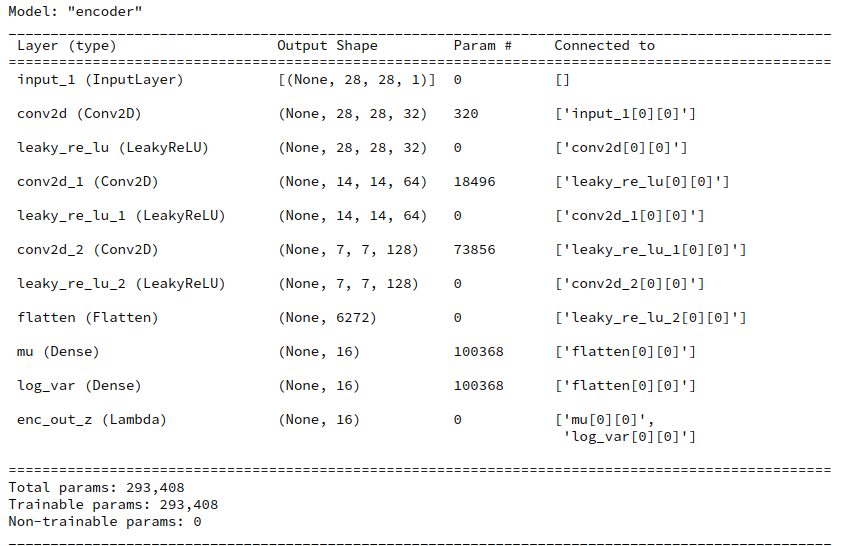The differences to the AE of the last post comprise two extra Dense layers: mu and log_var. Note that the dimension of the respective rank 1 tensor (a vector!) is equal to z_dim.

mu and log_var are (vector) variables which later shall be optimized. So, we have to treat them as trainable network variables. In the above code this is done via the weights of the two defined Dense layers which we integrated in the network. But note that we did not define any activation function for the layers! The calculation of “output” of these layers is done in a more complex function which we encapsulated in the function “sampling()”.
of
Therefore, we have in addition defined a Lambda layer which applies the (Lambda) function “sampling()” to the vectors mu and var_log. The Lambda layer thus delivers the required z-point (i.e. a vector) for each sample in the z-dimensional latent space.

# z-point calculation

How exactly do we calculate a z-point or vector in the latent space? And what does the KL loss, which we later shall use, punish? For details you need to read the literature. But in short terms:

Instead of calculating a z-point directly we use a Gaussian distribution depending on 2 parameters – our mean value “mu” and a standard deviation “var“. We calculate a statistically random z-point within the range of this distribution. Note that we talk about a vector-distribution. The distribution “variables” mu and log_var are therefore vectors.

If “x” is the last layer of our conventional network part then

```mu = Dense(self.z_dim, name='mu')(x)
log_var = Dense(self.z_dim, name='log_var')(x)
```

Having a value for mu and log_var we use a randomized factor “epsilon” to calculate a “variational” z-point with some statistical fluctuation:

```
epsilon = B.random_normal(shape=B.shape(mu), mean=0., stddev=1.)
z_point = mu + B.exp(log_var/2)*epsilon
```

This is the core of the “sampling()”-function which we defined a minute ago, already.

As you see the randomization of epsilon assumes a standard Gaussian distribution of possible values around a mean = 0 with a standard-deviation of 1. The calculated z-point is then placed in the vicinity of a fictitious z-point “mu”. The coordinate of mu” and the later value for log_var are subjects of optimization. More specifically, the distance of mu to the center of the latent space and too big values of the standard-deviation around mu will be punished by the loss. Mathematically this is achieved by the KL loss (see below). It will help to get a compact arrangements of the z-points for the training samples in the z-space (= latent space).

To transform the result of the above calculation into an ordinary z-point output of the Encoder for a given input sample we apply a Keras Lambda layer which takes the mu- and log_var-layers as input and applies the function “sampling()“, which we have already defined, to input in form of the mu and var_log tensors.

# The Decoder

The Decoder is pretty much the same as the on which we have defined earlier for the Autoencoder.

```dec_inp_z = Input(shape=(z_dim))
x = Dense(np.prod(shape_before_flattening))(dec_inp_z)
x = Reshape(shape_before_flattening)(x)
x = Conv2DTranspose(filters=64, kernel_size=3, strides=2, padding='same')(x)
x = LeakyReLU()(x)
x = Conv2DTranspose(filters=32, kernel_size=3, strides=2, padding='same')(x)
x = LeakyReLU()(x)
x = Conv2DTranspose(filters=1,  kernel_size=3, strides=1, padding='same')(x)
x = LeakyReLU()(x)
# Output -
x = Activation('sigmoid')(x)
dec_out = x
decoder = Model([dec_inp_z], [dec_out], name="decoder")
decoder.summary()
```# The Kullback-Leibler loss

Now, we must define a loss which helps to optimize the weights associated with the mu and var_log layers. We will choose the so called “Kullback-Leibler [KL] loss” for this purpose. Mathematically this convex loss function is derived from a general distance metric (Kullback_Leibler divergence) for two probability distributions. See:
https://en.wikipedia.org/ wiki/ Kullback-Leibler_divergence – and especially the examples section there
https://en.wikipedia.org/ wiki/ Divergence_(statistics)

In our case our target distribution we pick a normal distribution calculated from a specific set of vectors mu and log_var as the first distribution and compare it to a standard normal distribution with mu = 0.0 and standard deviation sqrt(exp(log_var) = 1.0. All for a specific z-point (corresponding to an input sample).
amp;
Written symbolically the KL loss is calculated by:

```kl_loss  = -0.5* mean(1 + log_var - square(mu) - exp(log_var))
```

The “mean()” accounts for the fact that we deal with vectors. (At this point not with respect to batches.) Our loss punishes a “mu” far off the origin of the “latent space” by a quadratic term and a more complicated term dependent on log_var and var = square(sigma) with sigma being the standard deviation. The loss term for sigma is convex around sigma = 1.0. Regarding batches we shall later take a mean of the individual samples’ losses.

This means that the KL loss tries to push the mu for the samples towards the center of the latent space’s origin and keep the variance around mu within acceptable limits. The mu-dependent term leads to an effective use of the latent space around the origin. Potential clusters of similar samples in the z-space will have centers not too far from the origin. The data shall not spread over vast regions of the latent space. The reduction of the spread around a mu-value keeps similar data-points in close vicinity – leading to rather confined clusters – as good as possible.

What are the consequences for the calculation of the gradient components, i.e. partial derivatives with respect to the individual weights or other trainable parameters of the ANN?

Note that the eventual values of the mu and log_var vectors potentially will depend

• on the KL loss and its derivatives with respect to the elements of the mu and var_log tensors
• the dependence of the mu and var_log tensors on contributory sums, activations and weights of previous layers of the Encoder
• and a sequence of derivatives defined by
• a loss function for differences between reconstructed output of the decoder in comparison to the encoders input,
• the decoder’s layers’ sum like contributions, activation functions and weights
• the output layer (better its weights and activation function) of the Encoder
• the sum-like contributions, weights and activation function of the Encoder’s layers

All partial derivatives are coupled by the “chain rule” of differential calculus and followed through the layers – until a specific weight or trainable parameter in the layer sequence is reached. So we speak about a mix, more precisely of a sum of two loss contributions:

• a reconstruction loss [reco_loss]: a standard loss for the differences of the output of the VAE with respect to its input and its dependence on all layers’ activations and weights. We have called this type of loss which measures the reconstruction accuracy of samples from their z-data the “reconstruction loss” for an AE in the last post. We name the respective variable “reco_loss”.
• Kullback-Leibler loss [kl_loss]: a special loss with respect to the values of the mu and log-var tensors and its dependence on previous layers’ activations and weights. We name the variable

The two different losses can be combined using weight factors to balance the impact of good reconstruction of the original samples and a confined distribution of data points in the z-space.

```total_loss = reco_loss + fact * kl_loss
```

I shall use the “binary_crossentropy” loss for the reco_loss term as it leads to good and fast convergence during training.

```reco_loss =>  binary_crossentropy
```

The total loss is a sum of two terms, but the corrections of the VAE’s network weights from either term by partial derivatives during error backward propagation affect different parts of the VAE: The optimization of the KL-loss directly affects only encoder weights, in our example of the mu-, the var_log- and the Conv2D-layers of the Encoder. The weights of the Decoder are only indirectly influenced by the KL-loss. The optimization of the “reconstruction loss” instead has a direct impact on all weights of all layers.

A VAE, therefore, is an interesting example for loss contributions depending on certain layers, only, or specific parts of a complex, composite ANN model with sub-divisions. So, the consequences of a rigorous “eager execution mode” of the present Tensorflow versions for gradient calculations are of real and general interest also for other networks with customized loos contributions.

# How to implement the KL-loss within a VAE-model? Some typical (older) methods ….

We could already define a (preliminary) keras model comprising the Encoder and the Decoder:

```enc_output = encoder(encoder_input)
decoder_output = decoder(enc_output)
vae_pre = Model(encoder_input, decoder_output, name="vae_witout_kl_loss")
```

This model would, however, not include the KL-loss. We, therefore, must make changes to it. I found four different methods in introductory ML, where most authors use the Keras functional interface to TF2 (however early versions) to set up a layer structure similar to ours above. See the references to the books in the final section of this post. Most authors the handle the KL-loss by using the tensors of our two special (Dense) layers for “mu” and “log_var” in the Encoder somewhat later:

• Method 1: D. Foster first creates his VAE-model and then uses separate functions to calculate the KL loss and a MSE loss regarding the difference between the output and input tensors. Afterward he puts a function (e.g. total_loss()) for adding both contribution up to a total loss into the Keras compile function as a closure – as in “compile(optimizer=’adam’, loss=total_loss)”.
• Method 2: F. Chollet in his (somewhat older) book on “Deep Learning with Keras and Python” defines a special customized Keras layer in addition to the Encoder and Decoder models of the VAE. This layer receives an internal function to calculate the total loss. The layer is then used as a final layer to define the VAE model and invokes the loss by the Layer.add_loss() functionality.
• Method 3: A. Geron in his book on “Machine Learning with Scikit-Learn, Keras and Tensorflow” also calculates the KL-loss after the definition of the VAE-model, but associates it with the (Keras) Model by the “model.add_loss()” functionality.
He then calls the models compile statement with the inclusion of a “binary_crossentropy” loss as the main loss component. As in
Geron’s approach relies on the fact that a loss defined by Model.add_loss() automatically gets added to the loss defined in the compile statement behind the scenes. Geron directly refers to the mu and log_var layers’ tensors when calculating the loss. He does not use an intermediate function.
• Method 4: A. Atienza structurally does something similar as Geron, but he calculates a total loss, adds it with model.add_loss() and then calls the compile statement without any loss – as in compile(optimzer=’adam’). Also Atienza calculates the KL-loss by directly operating on the layer’s tensors.

# A specific way of including the VAE loss (adaption of method 2)

A code snippet to realize method 2 is given below. First we define a class for a “Customized keras Layer”.

Customized Keras layer class:

```class CustVariationalLayer (Layer):

def vae_loss(self, x_inp_img, z_reco_img):
# The references to the layers are resolved outside the function
x = B.flatten(x_inp_img)   # B: tensorflow.keras.backend
z = B.flatten(z_reco_img)

# reconstruction loss per sample
# Note: that this is averaged over all features (e.g.. 784 for MNIST)
reco_loss = tf.keras.metrics.binary_crossentropy(x, z)

# KL loss per sample - we reduce it by a factor of 1.e-3
# to make it comparable to the reco_loss
kln_loss  = -0.5e-4 * B.mean(1 + log_var - B.square(mu) - B.exp(log_var), axis=1)
# mean per batch (axis = 0 is automatically assumed)
return B.mean(reco_loss + kln_loss), B.mean(reco_loss), B.mean(kln_loss)

def call(self, inputs):
inp_img = inputs
out_img = inputs
total_loss, reco_loss, kln_loss = self.vae_loss(inp_img, out_img)

return out_img  #not really used in this approach
```

Now, we add a special layer based on the above class and use it in the definition of a VAE model. I follow the code in F. Cholet’s book; there the customized layer concludes the layer structure after the Encoder and the Decoder:

```enc_output = encoder(encoder_input)
decoder_output = decoder(enc_output)

# add the custom layer to the model
fc = CustVariationalLayer()([encoder_input, decoder_output])

vae = Model(encoder_input, fc, name="vae")
vae.summary()
```

The output fits the expectations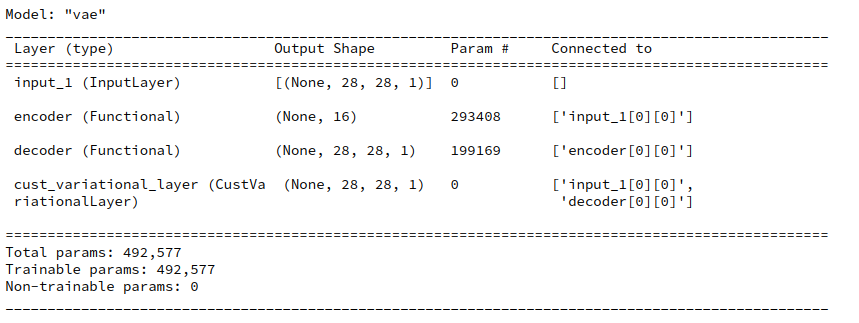Eventually we need to compile. F. Chollet in his book from 2017 defines the loss as “None” because it is covered already by the layer.

```vae.compile(optimizer=Adam(), loss=None)
```

# fit() fails … a typical error message

We are ready to train – at least I thought so … We start training with scaled x_train images coming e.g. from the MNIST dataset by the following statements :

```n_epochs = 3;
batch_size = 128
vae.fit( x=x_train, y=None, shuffle=True,
epochs = n_epochs, batch_size=batch_size)
```

Unfortunately we get the following typical

Error message:

```
TypeError: You are passing KerasTensor(type_spec=TensorSpec(shape=(), dtype=tf.float32, name=None), name='tf.math.reduce_sum_1/Sum:0', description="created by layer 'tf.math.reduce_sum_1'"), an intermediate Keras symbolic input/output, to a TF API that does not allow registering custom dispatchers, such as `tf.cond`, `tf.function`, gradient tapes, or `tf.map_fn`. Keras Functional model construction only supports TF API calls that *do* support dispatching, such as `tf.math.add` or `tf.reshape`. Other APIs cannot be called directly on symbolic Kerasinputs/outputs. You can work around this limitation by putting the operation in a custom Keras layer `call` and calling that layer on this symbolic input/output.
```

# The error is due to “eager execution”!

The problem I experienced was related to a concise “eager mode execution” implemented in Tensorflow ≥ 2.3. If we deactivate “eager execution” by the following statement before we define any layers

```from tensorflow.python.framework.ops import disable_eager_execution
disable_eager_execution()
```

then we get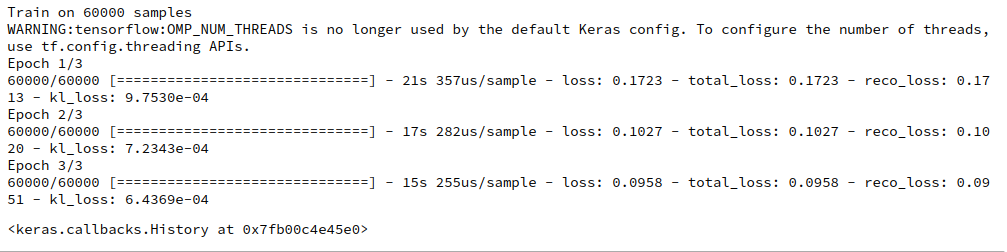You can safely ignore the warning which is due to a superfluous environment variable.

# And the other approaches for the KL loss?

Addendum 25.05.2022: This paragraph had to be changed as its original version contained wrong statements.

I had and have code examples for all the four variants of implementing the KL loss listed above. Both methods 1 and 2 do NOT work with standard TF 2.7 or 2.8 and active “eager execution” mode. Similar error messages as described for method 2 came up when I tried the original code of D. Foster, which you can download from his github repository. However, methods 3 and 4 do work – as long as you avoid any intermediate function to calculate the KL loss.

Important remark about F. Chollet’s present solution:
I should add that F. Chollet has, of course, meanwhile published a modern and working approach in the present online Keras documentation. I shall address his actual solution in a later post. His book is also the oldest of the four referenced.

# Conclusion

In this post we have invested a lot of effort to realize a Keras model for a VAE – including the Kullback-Leibler loss. We have followed recipes of various books on Machine Learning. Unfortunately, we have to face a challenge:

Recipes of how to implement the KL loss for VAEs in books which are older than ca. 2 years may not work any longer with present versions of Tensorflow 2 and its “eager execution mode”.

In the next post of this series
Variational Autoencoder with Tensorflow 2.8 – IV – simple rules to avoid problems with eager execution
we shall have a closer look at the problem. I will try to derive a central rule which will be helpful for the design various solutions that do work with TF 2.8.

# Literature references

F. Chollet, Deep Learning mit Python und Keras, 2018, 1-te dt. Auflage, mitp Verlags GmbH & Co.KG, Frechen

D. Foster, “Generatives Deep Learning”, 2020, 1-te dt. Auflage, dpunkt Verlag, Heidelberg in Kooperation mit Media Inc.O’Reilly, ISBN 978-3-960009-128-8. See Kap. 3 and the VAE code published at

A. Geron, “Hands-On Machine Learning with Scikit-Learn, keras & Tensorflow”, 2019, 2nd edition, O’Reilly, Sebastpol, Canada, ISBN 978-1-492-03264-9. See chapter 17.

R. Atienza, “Advanced Deep Learniing with Tensorflow 2 and Keras, 2020, 2nd edition, Packt Publishing, Birminham, UK, ISBN 978-1-83882-165-4. See chapter 8.

Ceterum censeo: The worst living fascist and war criminal today, who must be isolated, denazified and imprisoned, is the Putler.

# Variational Autoencoder with Tensorflow 2.8 – II – an Autoencoder with binary-crossentropy loss

In the last post of this series

Variational Autoencoder with Tensorflow 2.8 – I – some basics

I have briefly discussed the basic elements of a Variational Autoencoder [VAE]. Among other things we identified an Encoder, which transforms sample data into z-points in a low dimensional “latent space”, and a Decoder which reconstructs objects in the original variable space from z-points.

In the present post I want to demonstrate that a simple Autoencoder [AE] works as expected with Tensorflow 2.8 [TF2]. In contrast to certain versions of Variational Autoencoders which we shall test in the next post. For our AE we use the “binary cross-entropy” as a suitable loss to compare reconstructed MNIST images with the original ones.

# A simple AE

I use the functional Keras API to set up the Autoencoder. Later on we shall encapsulate everything in a class, but lets keep things simple for the time being. We design both the Encoder and the Decoder as CNNs and use properly configured Conv2D and Conv2DTranspose layers as their basic elements.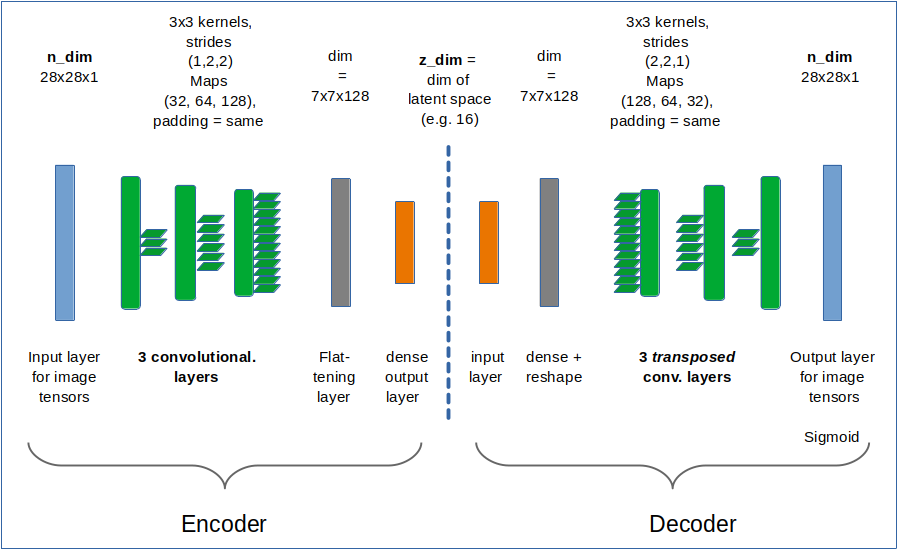Required Imports

```# tensorflow and keras
import tensorflow as tf
from tensorflow import keras as K
from tensorflow.keras import backend as B
from tensorflow.keras.models import Model
from tensorflow.keras import regularizers
from tensorflow.keras import metrics
from tensorflow.keras.layers import Input, Conv2D, Flatten, Dense, Conv2DTranspose, Reshape, Lambda, \
Activation, BatchNormalization, ReLU, LeakyReLU, ELU, Dropout, \

from tensorflow.keras.utils import to_categorical
from tensorflow.keras.optimizers import schedules
```

z_dim and the Encoder defined with the functional API
We set the dimension of the “latent space” to z-dim = 16.
This allows for a very good reconstruction of images in the MNIST case.

```z_dim = 16
encoder_input = Input(shape=(28,28,1)) # Input images pixel values are scaled by 1./255.
x = encoder_input
x = Conv2D(filters = 32, kernel_size = 3, strides = 1, padding='same')(x)
x = LeakyReLU()(x)
x = Conv2D(filters = 64, kernel_size = 3, strides = 2, padding='same')(x)
x = LeakyReLU()(x)
x = Conv2D(filters = 128, kernel_size = 3, strides = 2, padding='same')(x)
x = LeakyReLU()(x)
shape_before_flattening = B.int_shape(x)[1:]  # B: Keras backend
x = Flatten()(x)
encoder_output = Dense(self.z_dim, name='encoder_output')(x)
encoder = Model([encoder_input], [encoder_output], name="encoder")
```

This is almost an overkill for something as simple as MNIST data. The Decoder reverses the operations. To do so it uses Conv2DTranspose layers.

The Decoder

```dec_inp_z      = Input(shape=(z_dim))
x = Dense(np.prod(shape_before_flattening))(dec_inp_z)
x = Reshape(shape_before_flattening)(x)
x = Conv2DTranspose(filters=64, kernel_size=3, strides=2, padding='same')(x)
x = LeakyReLU()(x)
x = Conv2DTranspose(filters=32, kernel_size=3, strides=2, padding='same')(x)
x = LeakyReLU()(x)
x = Conv2DTranspose(filters=1,  kernel_size=3, strides=1, padding='same')(x)
x = LeakyReLU()(x)
# Output into a region of 0 to 1 - requires a one hot encoding of classes
# and scaling of pixel values of the input samples to [0, 1]
x = Activation('sigmoid')(x)
decoder_output = x
decoder = Model([dec_inp_z], [decoder_output], name="decoder")
```

The full AE-model

```ae_input  = encoder_input
ae_output = decoder(encoder_output)
AE = Model(ae_input, ae_output, name="AE")
```

That was easy! Now we compile:

```AE.compile(optimizer=Adam(learning_rate = 0.0005), loss=['binary_crossentropy'])
```

I use the “binary_crossentropy” loss function to evaluate and punish differences between the reconstructed image and the original. Note that we need to scale pixel values of input images (as MNIST images) down to a value range between [0, 1] due to using a sigmoid activation function at the output layer of the Decoder.
Using “binary cross-entropy” leads to a better and faster convergence of the training process:

# Training and results of the Autoencoder for MNIST samples

Eventually, we can train our model:

```n_epochs = 40
batch_size = 128
AE.fit( x=x_train, y=x_train, shuffle=True,
epochs = n_epochs, batch_size=batch_size)
```

After 40 training steps we can visualize the resulting data clusters in z-space. To get a 2-dimensional plot out of 16-dimensional data requires the use of t-SNE. For 15.000 training data (out of 60.000) we get:

Our Encoder CNN was able to separate the different classes quite well by extraction basic features of the handwritten digits by its Conv2D layers. The test which the AE never had seen before are well separated too:

Note that the different position of the clusters on the 2-dim plots are due to transformations and arrangements of t-SNE and have no deeper meaning.

For z_dim = 16 it is almost perfect: Below you see a sequence of rows presenting original images and their reconstructed counterparts for selected MNIST test data, which the AE had not seen before: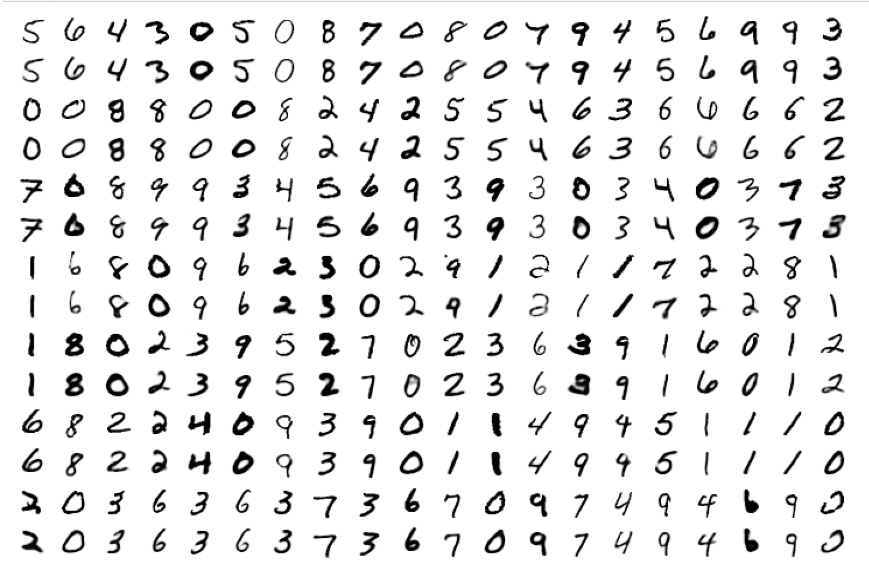# The special case of “z_dim = 2”

When we set z_dim = 2 the reconstruction quality of course suffers: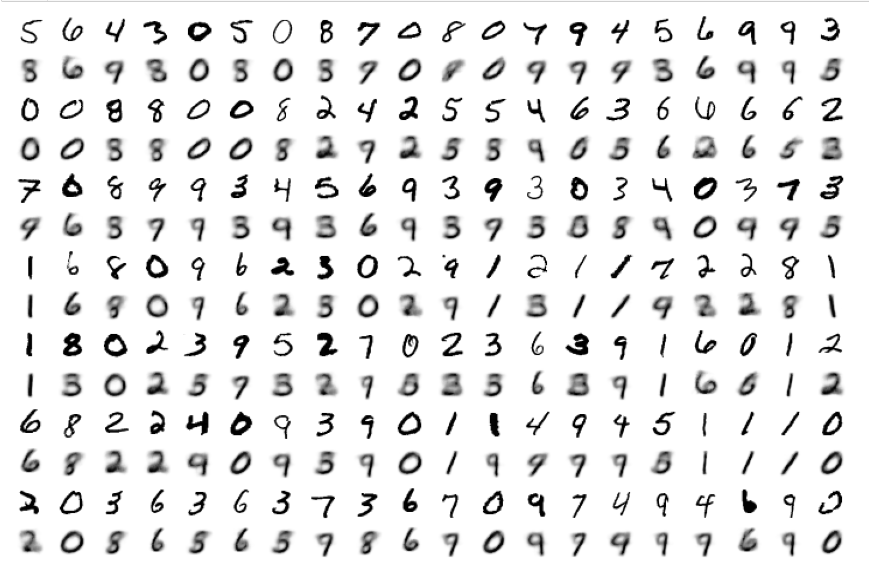Regarding class related clusters we can directly plot the data distributions in the z-space: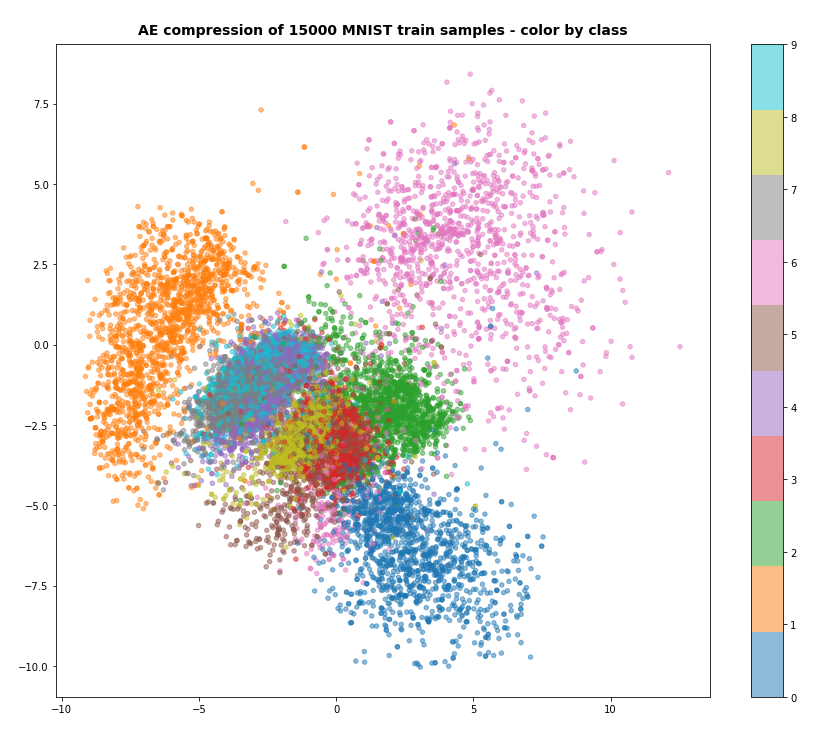We see a relatively vast spread of data points in clusters for “6”s and “0”s. We also recognize the typical problem zones for “3”s, “5”s, “8”s on one side and for “4”s, “7”s and “9”s on the other side. They are difficult to distinguish with only 2 dimensions.

# Conclusion

Autoencoders are simple to set up and work as expected together with Keras and Tensorflow 2.8.

In the next post

Variational Autoencoder with Tensorflow 2.8 – III – problems with the KL loss and eager execution

we shall, however, see that VAEs and their KL loss may lead to severe problems.

Ceterum censeo: The worst living fascist and war criminal today, who must be isolated, denazified and imprisoned, is the Putler.

# Variational Autoencoder with Tensorflow 2.8 – I – some basics

Last week I tried to perform some systematic calculations with a “Variational Autoencoder” [VAE] for a presentation about Machine Learning [ML]. I wanted to apply VAEs to different standard datasets (MNIST Fashion, CIFAR 10, Celebrity) and demonstrate some basic capacities of VAE algorithms. A standard task … well covered by several introductory books on ML.

Actually, I had some 2 years old Python modules for VAEs available – all based on recommendations in the literature. My VAE-model setup had been done with Keras. Moreprecisely the version integrated into Tensorflow 2 [TF2]. But I ran into severe trouble with my present Tensorflow versions, namely 2.7 and 2.8. The cause of the problems was my handling of the so called Kullback-Leibler [KL] loss in combination with the “eager execution mode” of TF2.

Actually, the problems may already have arisen with earlier TF versions. With this post series I hope to save time for some readers who, as myself, are no ML professionals. In a first post I will briefly repeat some basics about Autoencoders [AEs] and Variational Autoencoders [VAEs]. In a second post we shall build a simple Autoencoder. In a third post I shall turn to VAEs and discuss some variants for dealing with the KL loss – all variants will be picked from recipes of ML teaching books. I shall demonstrate afterward that some of these recipes fail for TF 2.8. A fourth post will discuss the problem with “eager execution” and derive a practical rule for calculations based on layer specific data. In three further posts I apply the rule in the form of three different variants for the KL loss of VAE models. I will set up the VAE models with the help of the functional Keras API. The suggested solutions all do work with Tensorflow 2.7 and 2.8.

If you are not interested in the failures of classical approaches to the KL loss presented in teaching books on ML and if you are not at all interested in theory you may skip the first four posts – and jump to the solutions.

# Some basics of VAEs – a brief introduction

I assume that the reader is already familiar with the concepts of “Variational Autoencoders”. I nevertheless discuss some elements below to get a common vocabulary.

I call the vector space which describes the input samples the "variable space". As AEs and VAEs are typically used for images this “variable space” has many dimensions – from several hundred up to millions. I call the number of these dimensions "n_dim".

An input sample can either be represented as a vector in the variable space or by a multi-ranked tensor. An image with 28×28 pixels and gray “colors” corresponds to a 784 dimensional vector in the variable space or a a “rank 2 tensor” with the shape (28, 28). I use the term “rank” to avoid any notional ambiguities regarding the term “dimensions”. Remember that people sometimes speak of 2-“dimensional” Numpy matrices with 28 elements in each dimension.

An Autoencoder [AE] typically consists of two connected artificial neural networks [ANN]:

• Encoder : maps a point of the “variable space” to a point in the so called “latent space”
• Decoder : maps a point of the “latent space” to a point in the “variable space”.

Both parts of an AE can e.g. be realized with Dense and/or Conv2D layers. See the next post for a simple example of a VAE layer-layout. With Keras both ANNs can be defined as individual “Keras models” which we later connect.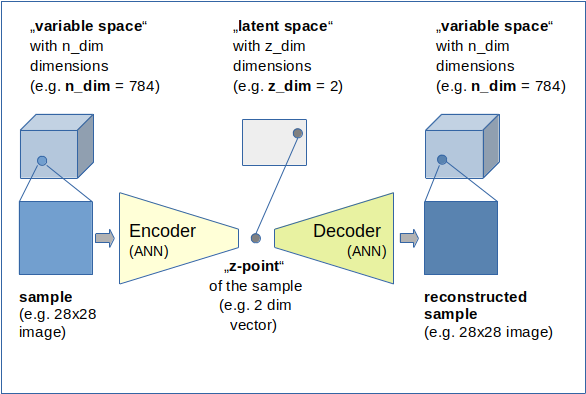Some more details:

• Encoder:
The Encoder ANN creates a vector for each input sample (mostly images) in a low-dimensional vector space, often called the “latent space“. I will sometimes use the abbreviation “z-space” for it and call a point in it a “z-point“. The dimensions of the “z-space” are given by a number “z_dim“. A “z-point” is therefore represented as a z-dimensional vector (a special kind of a tensor of rank 1). The Encoder realizes a non-linear transformation of n-dimensional vectors to z-dimensional vectors. Encoders, actually, are very effective data compressors. z_dim may take numbers between 2 and a few hundred – depending on the complexity of data and objects represented by the input samples with much higher dimensions (some hundreds to millions).
• Decoder:
The “Decoder” ANN instead takes arbitrary low dimensional vectors from the “latent space” as input and creates or better “reconstructs” tensors with the same rank and dimensions as the input samples. These output samples can, of course, also be represented by n-dimensional vectors in the original variable space. The Decoder is a kind of inversion of the “Encoder”. This is also reflected in its structure as we shall see later on. One objective of (V)AEs is that the reconstruction vector for a defined input sample should be pretty close to the input vector of the very same sample – with some metric to measure distances. In natural terms for images: An output image of a Decoder should be pretty comparable to the input image of the Encoder, when we feed the Decode with the z-dim vector created by the Encoder.

A Keras model for the (V)AE can be build by connecting the models for the Encoder and Decoder. The (V)AE model is trained as a whole to minimize differences between the input (e.g. an image) presented to the Encoder and the created output of the Decoder (a reconstructed image). A (V)AE is trained “unsupervised” in the sense that the “label data” for the training are identical to the encoder input samples.

# Encoder and Decoder as CNNs?

When you build the Encoder and the Decoder as a “Convolutional Neutral Networks” [CNNs] they support data compression and reconstruction very effectively by a parallel extraction of basic “features” of the input samples and putting the related information into neural “maps”. (The neurons in such a “map” react sensitively to correlation patterns of components of the tensors representing the input data samples.) For images such CNN networks would accept rank 2 tensors.

Note that the (V)AE’s knowledge about basic “features” (or data correlations) in the input samples is encoded in the weights of the “Encoder” and “Decoder”. The points in the “latent space” which represent the input samples may show cluster patterns. The clusters could e.g. be used in classification tasks. However, without an appropriate Decoder with fitted weights you will not be able to reconstruct a complete image from a point in the z-space. Similar to encryption scenarios the Decoder is a kind of “key” to retrieve original information (even with reduced detail quality).

# Variational Autoencoders

Variational Autoencoders take care about a reasonable data organization in the latent space – in addition to data compression. The objective is to get well defined and segregated clusters there for “features” or maybe even classes of input samples. But the clusters should also be confined in a common, relatively small and compact region of the z-space.

To achieve this goal one judges the arrangement of data points, i.e. their distribution in the latent space, with the help of a special loss contribution. I.e. we use specially designed costs which punish vast extensions of the data distribution off the origin in the latent space.

The data distribution is controlled by parameters, typically a mean value “mu” and a standard deviation or variance “var”. These parameters provide additional degrees of freedom during training. The special loss used to control the predicted distribution in comparison to an ideal one is the so called “Kullback-Leibler” [KL] loss. It measures the “distance” of the real data distribution in the latent space from a more confined ideal one.

You find more information about the KL-loss in the books of D. Foster and R. Atienza named in the last section of this post.

# Why the fuss about VAEs and the KL-loss at all?

Why are VAEs and their “KL loss” important? Basically, because VAEs help you to confine data in the z-space. Especially when the latent space has a very low number of dimensions. VAEs thereby also help to condense clusters which may be dominated by samples of a specific class or a label for some specific feature. Thus VAEs provide the option to define paths between such clusters and allow for “feature arithmetics” via vector operations.

See the following images of MNIST data mapped into a 2-dimensional latent space by a standard Autoencoder: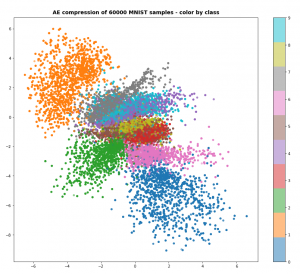Despite the fact that the AE’s sub-nets (CNNs) already deal very well with with features the resulting clusters for the digit classes are spread in a relatively large area of the latent space: [-7 ≤ x ≤ +7], [6 ≥ y ≥ -9]. Had we used dense layers of a MLP only, the spread of data points would have covered an even bigger area in the latent space.

The following three plots resulted from VAEs with different parameters. Watch the scale of the axes.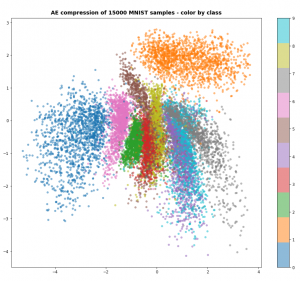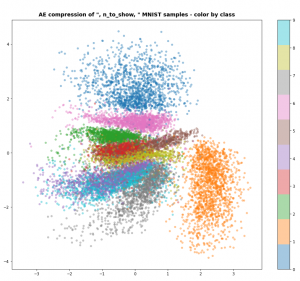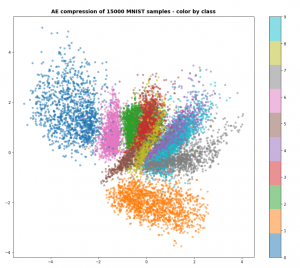The data are now confined to a region of around [[-4,4], [-4,4]] with respect to [x,y]-coordinates in the “latent space”. The available space is more efficiently used. So, you want to avoid any problems with the KL-loss in VAEs due to some peculiar requirements of Tensorflow 2.x.

# Conclusion

AEs are easy to understand, VAEs have subtle additional properties which seem to be valuable for certain tasks. But also for principle reasons we want to enable a Keras model of an ANN to calculate quantities which depend on the elements of one or a few given layers. One example of such a quantity is the KL loss.

In the next post

Variational Autoencoder with Tensorflow 2.8 – II – an Autoencoder with binary-crossentropy loss

we shall build an Autoencoder and apply it to the MNIST dataset. This will give us a reference point for VAEs later.

Stay tuned ….
Ceterum censeo: The worst living fascist and war criminal today, who must be isolated, denazified and imprisoned, is the Putler.